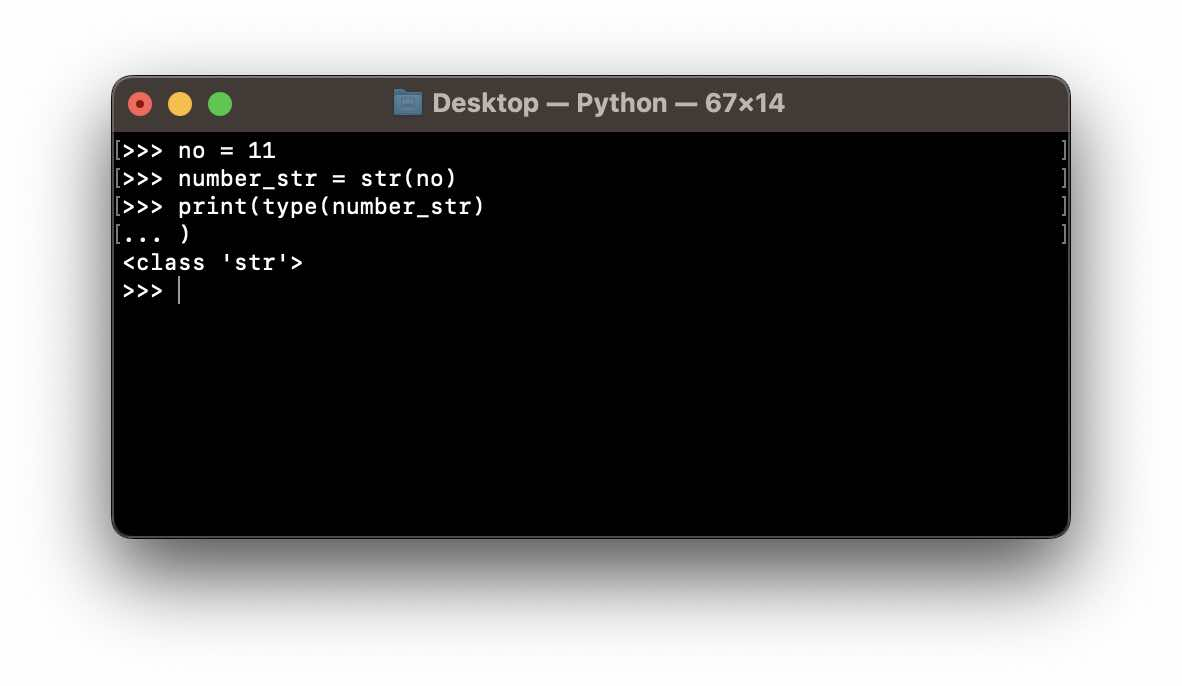# How to convert an int to a string in Python

We can convert an integer (int) to a String in Python by casting the int with str() function.

Example:

Let's say we have an integer with value 23.

``number = 23``

You can check the type of a data type by using the type() built-in function.

``print(type(number))``

<class 'int'>

Now to convert this integer to a string let's make use of the str function and save it as number_str.

``number_str = str(number)``

Again to verify, let's check the type of the variable number_str

``print(type(number_str))``

<class 'str'>

Complete Code:
``````int_number = 23

#conveting int to str
number_str = str(int_number)

print(f"type of int_number: {type(int_number)}")
print(f"type of number_str: {type(number_str)}")``````

type of int_number: <class 'int'>
type of number_str: <class 'str'>

class str(object=b'', encoding='utf-8', errors='strict')

Return a string version of object. If object is not provided, returns the empty string.

Built-in str() method: https://docs.python.org/3/library/stdtypes.html#str-

### Facing issues? Have Questions? Post them here! I am happy to answer!

Author Info:

Rakesh is a seasoned developer with over 10 years of experience in web and app development, and a deep knowledge of operating systems. Author of insightful How-To articles for Code2care.